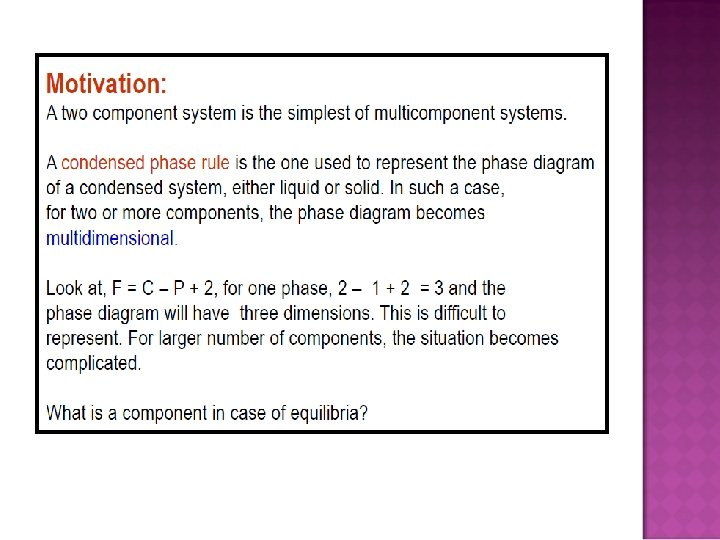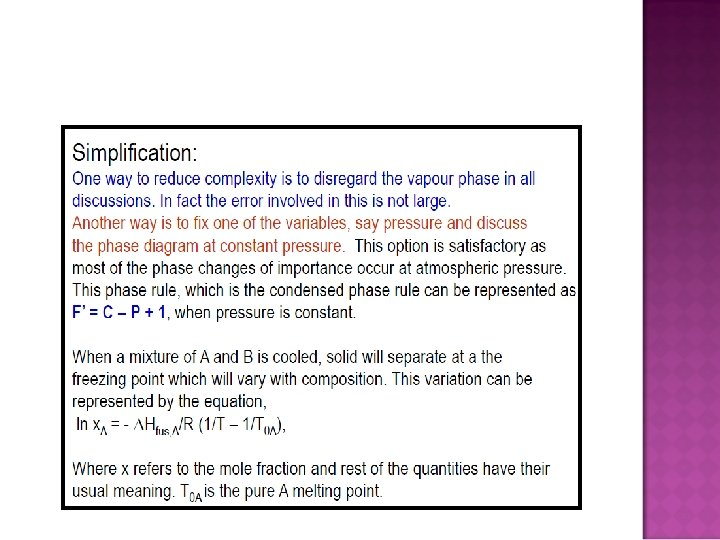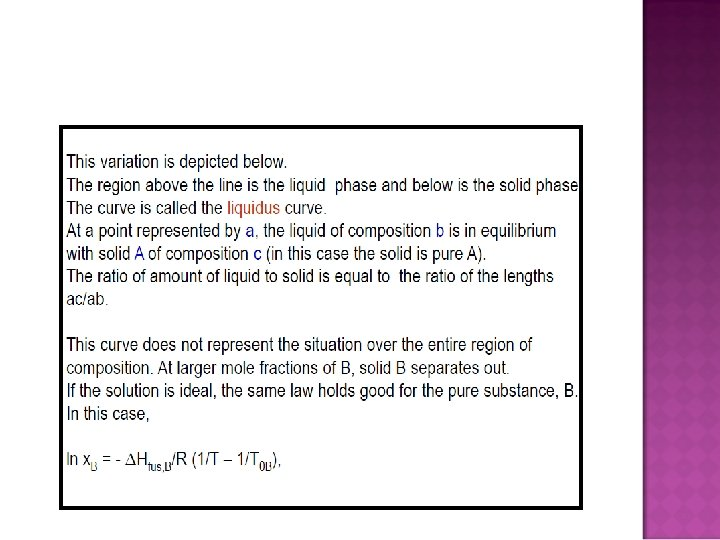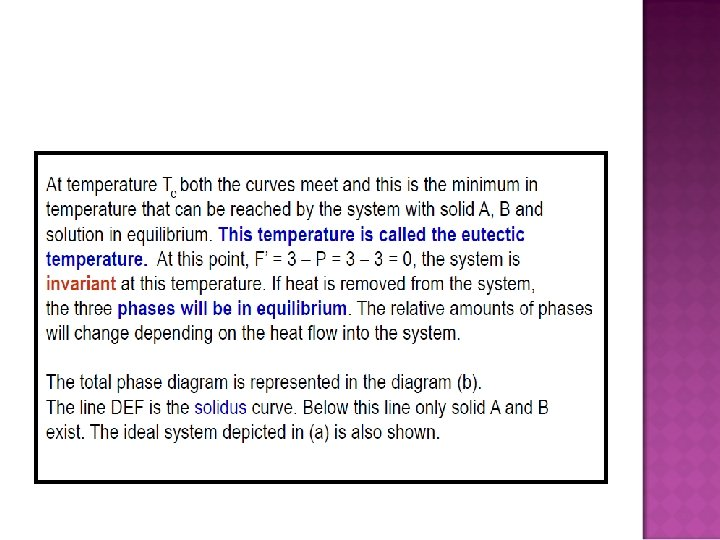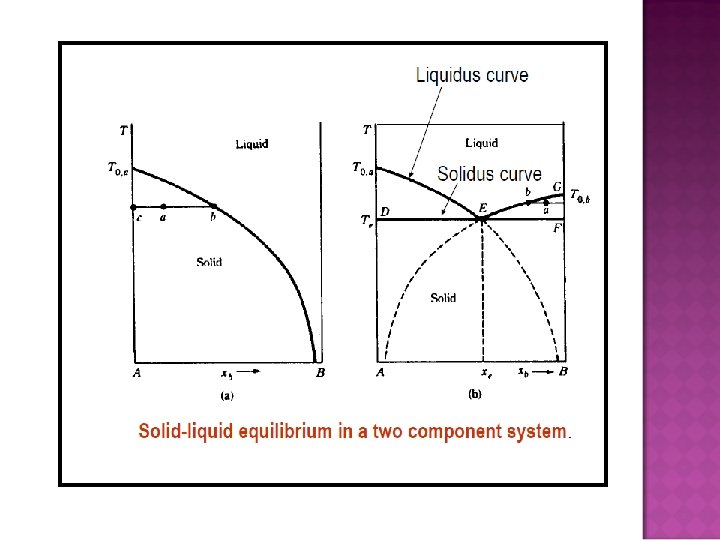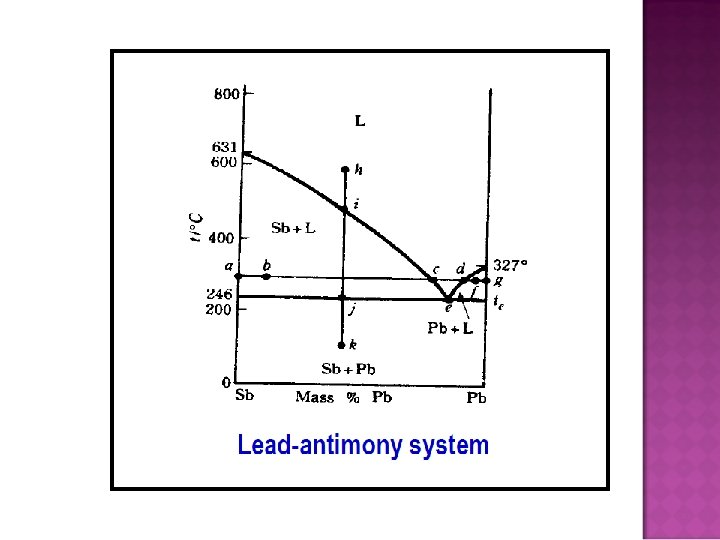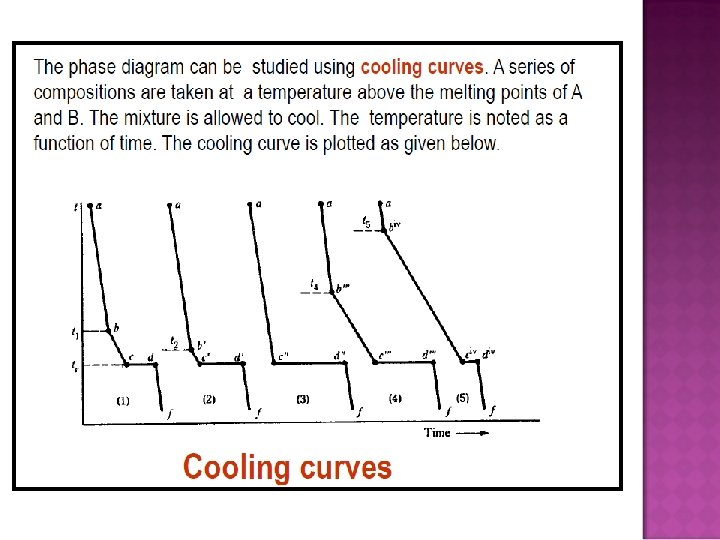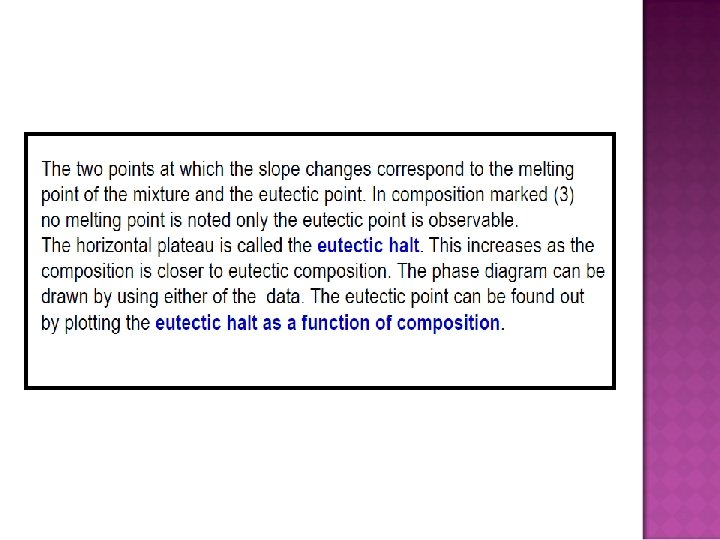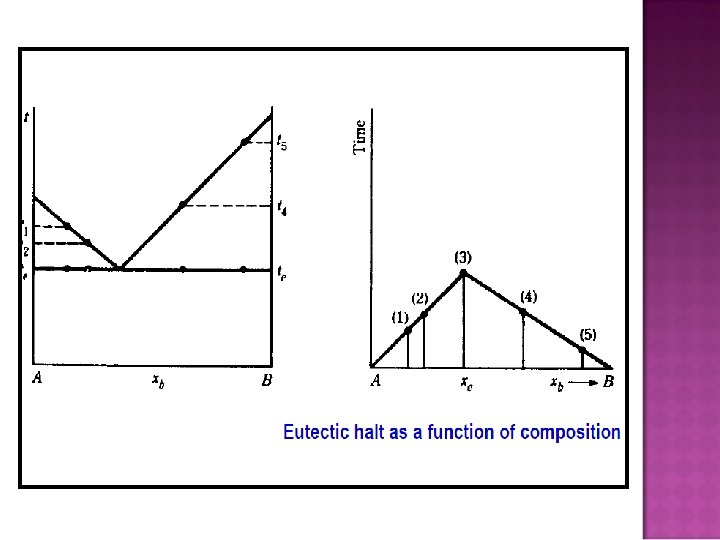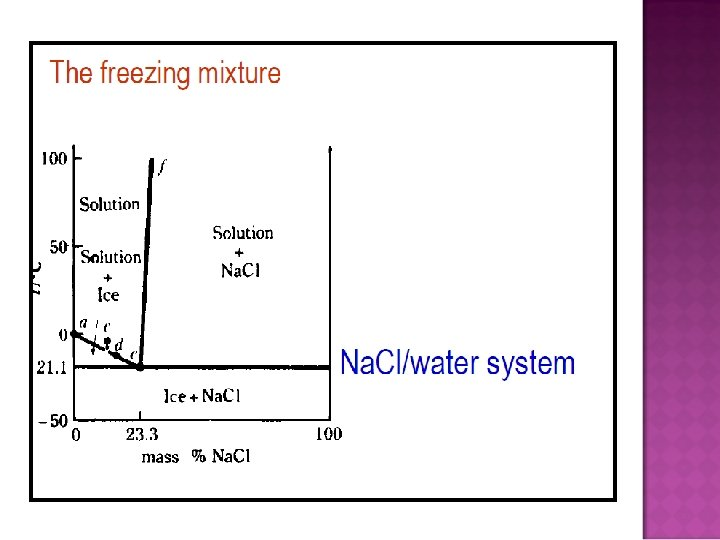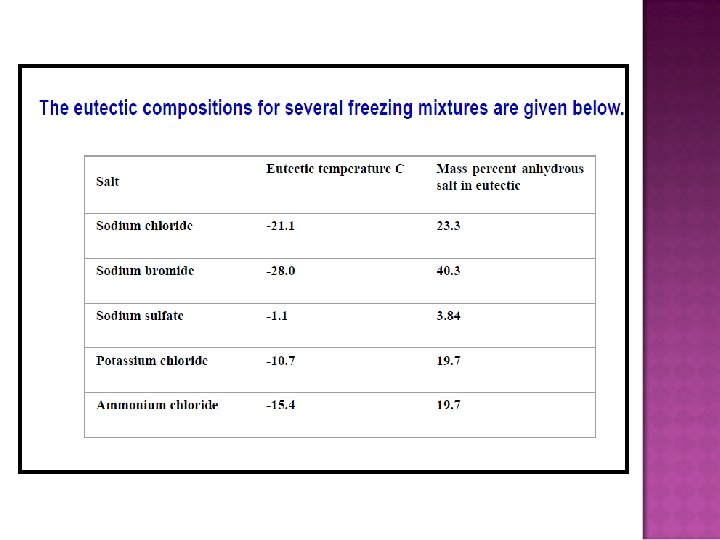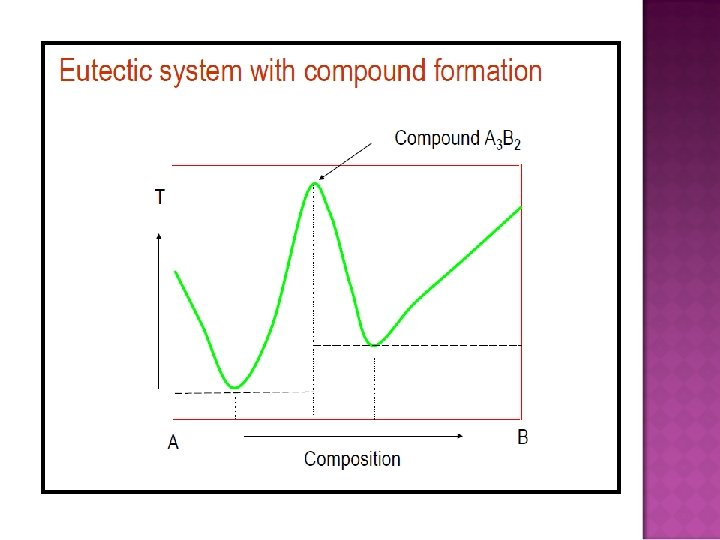# Why does an ice cube melt in the

• Slides: 32� Why does an ice cube melt in the mouth?PHASE DIAGRAMS � Phase: is a form of matter that is uniform throughout in chemical composition and physical state. � Phase transitions: the spontaneous conversion of one phase into another phase, occurs at a characteristic temperature for a given pressure. � Transition temperature: the temperature at which the two phases are in equilibrium and the Gibbs energy of the system is minimized at the prevailing pressure.�A phase is a component within a system, existing in a precisely defined physical state, e. g. gas, liquid, or a solid that has a single crystallographic form. � A phase diagram is a graph showing values of applied pressure and temperature at which equilibrium exists. � A phase boundary is a line on a phase diagram representing values of applied pressure and temperature at which equilibrium exists.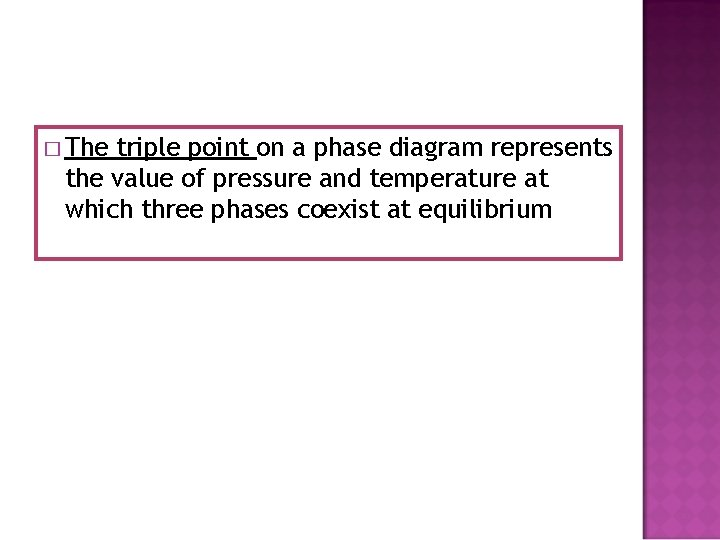� The triple point on a phase diagram represents the value of pressure and temperature at which three phases coexist at equilibrium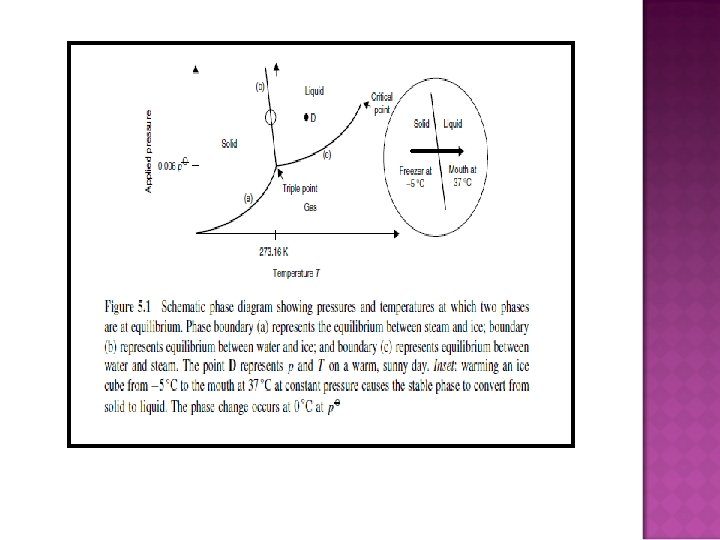PRESSURE AND TEMPERATURE CHANGES WITH A SINGLE-COMPONENT SYSTEM: QUALITATIVE DISCUSSION Effect of temperature on a phase change: sublimation � How � Dry is the ‘Smoke’ in horror films made? ice is solid carbon dioxide.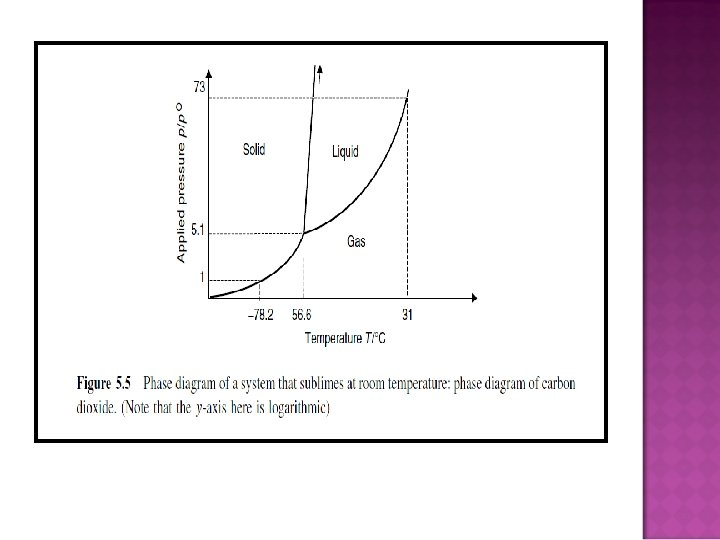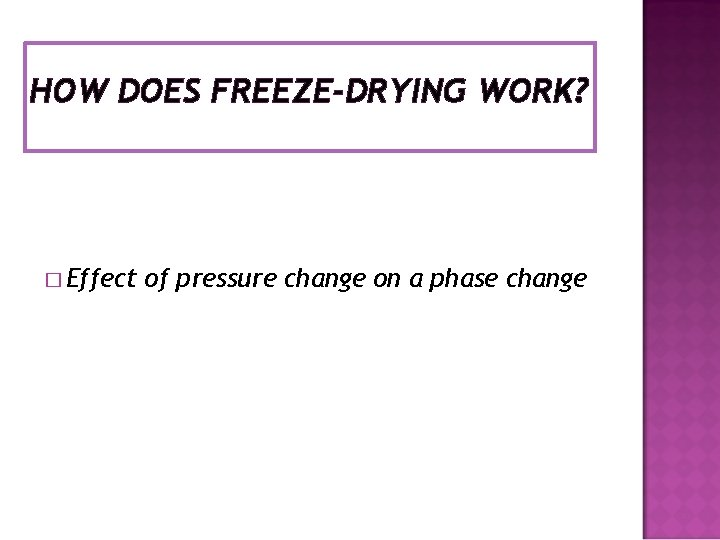HOW DOES FREEZE-DRYING WORK? � Effect of pressure change on a phase change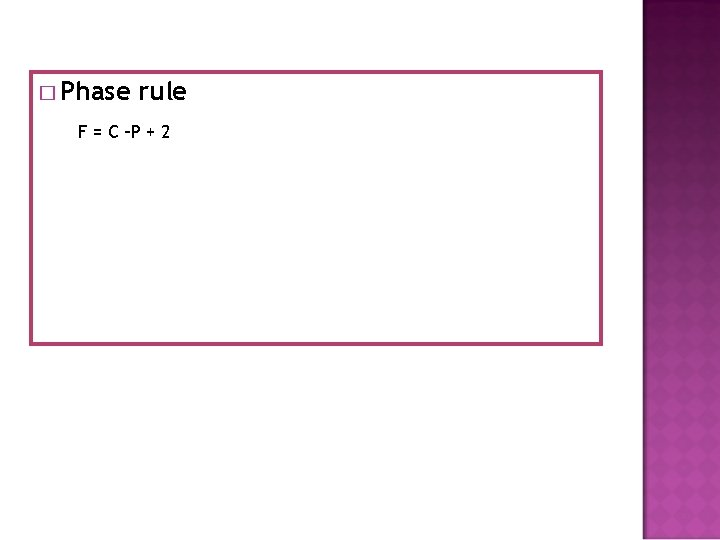� Phase rule F = C –P + 2